Back-end Engineering Articles

I write and talk about backend stuff like Ruby, Ruby On Rails, Databases, Testing, Architecture / Infrastructure / System Design, Cloud, DevOps, Backgroud Jobs, and more...

@daniel_moralesp

2019-05-03

## Control Flow and Conditionals in Ruby

This is one of the critical things you have to understand very well to become a good developer. This is an extensive topic, so we'll need to explain many things, but the first and most important one is a control flow.

# Control flow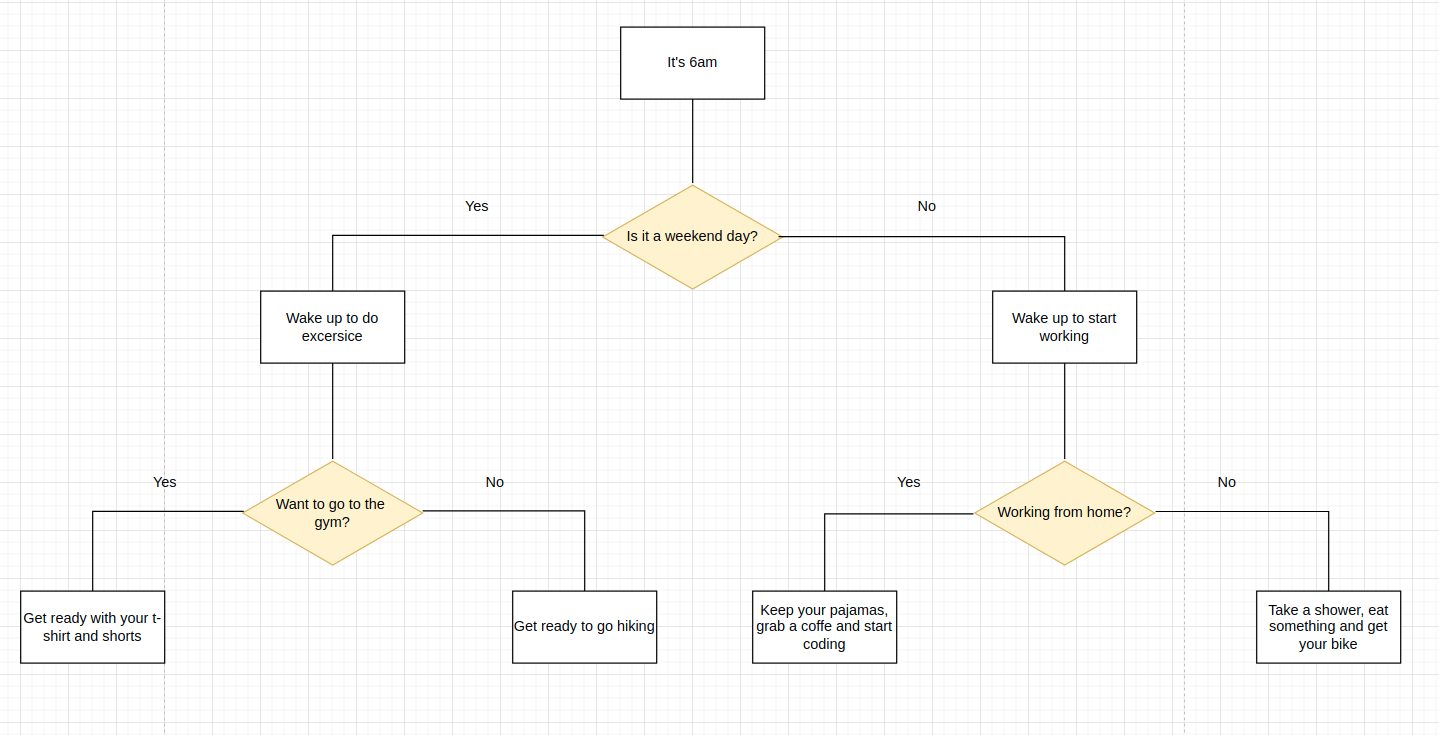Note: you don't have to wake up on the weekend at 6am, just an example :)

Control flow is a series of steps taken according to an answer or conditional. The conditionals are given inside the yellow isometric square in the image above. These conditionals are constantly evaluated as true or false (in our case, as Yes or No). That's the building block of almost all the code you'll be writing at the very beginning. After that, you'll start evaluating conditionals and doing something accordingly.

This also seems like a decision tree, and actually, it is a decision tree. According to the answers given to the question (conditional), you are falling down. What we need to do here is to translate this diagram to Ruby code. But before that, let's see another vital concept: Comparison operators.

# Comparison Operators

In one of the last blog posts, Ruby Data Types: Strings, Numbers, and Booleans, we studied Booleans. Remember that: "The Boolean data type is primarily associated with conditional statements, which allow different actions by changing control flow depending on whether a programmer-specified Boolean condition evaluates to true or false. It is a special case of a more general logical data type (see probabilistic logic)—logic doesn't always need to be Boolean."

Let's check this concept in our IRB Console again.

```2.6.8 :016 > true
=> true
2.6.8 :017 > false
=> false
2.6.8 :018 > true.class
=> TrueClass
2.6.8 :019 > false.class
=> FalseClass ```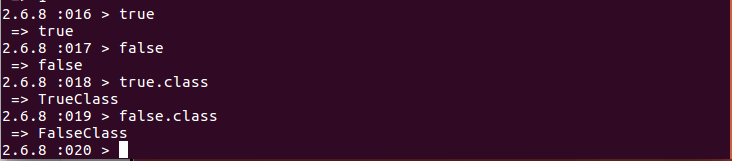We can create Boolean expressions using comparison operators. Comparison operators compare two items and return True or False. They are also known as "relational." The first two comparison operators that we will see here are:

• * Equal: ==
• * Not equal: !=

Let's do a couple of examples.

```2.3.3 :001 > 1 == 1
=> true
2.3.3 :002 > 2 != 4
=> true
2.3.3 :003 > 3 == 5
=> false
2.3.3 :004 > '7' == 7
=> false ```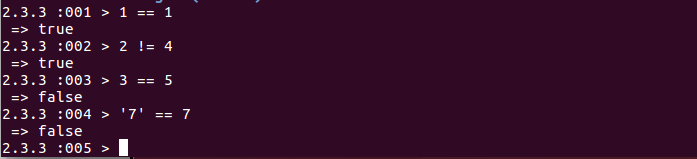As we can see, now we're not using just one equal sign "=" because it helps us assign a value to a variable, as we saw in the last blog post. What we're doing now is a comparison, asking the machine if:

• * “1 is equal to 1” or 1 == 1 which is true
• * "2 is not equal to 4" or 2 != 4 which is true
• * “3 is equal to 5” or 3 == 5 which is false
• * "7 as a string is equal to 7 as a number" or '7' == 7, which is false. This one is a little tricky because we are comparing two different data types; the first one is a string while the second one is an integer; for that reason, the result is false.

These lines of code can be read as a question or with a question mark at the end. But, of course, you always have to have a true or false result, not something ambiguous.

# Boolean variables

This is any variable with a boolean value assigned to it, such as True or False, either directly or because it will evaluate some condition. Let's make a couple of examples.

```2.3.3 :015 > set_to_true = true
=> true
2.3.3 :016 > set_to_true
=> true
2.3.3 :017 > set_to_false = false
=> false
2.3.3 :018 > set_to_false
=> false
2.3.3 :019 > bool_one = 5 != 7
=> true
2.3.3 :020 > bool_one
=> true
2.3.3 :021 > bool_two = 1 + 1 != 2
=> false
2.3.3 :022 > bool_two
=> false
2.3.3 :023 > bool_three = 3 * 3 == 9
=> true
2.3.3 :024 > bool_three
=> true ```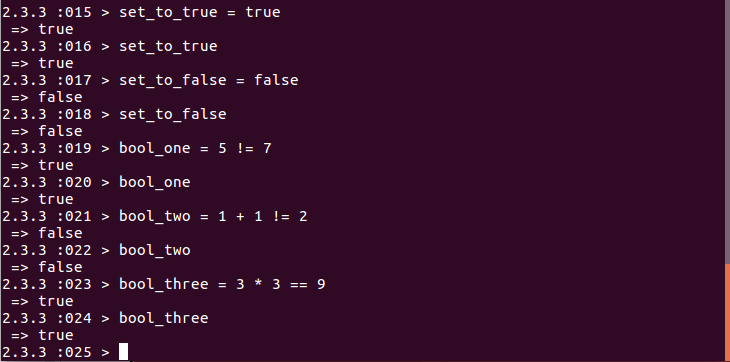We're now doing things a little bit more complicated than before.

• * First lines of code assign values true or false directly to a variable
• * Then we have bool_one who has a comparison operator "!=". Seems a little awkward because we have the equal sign "=" to assign to the variable, and then we have the "not equal" expression. If we print the result, we have a Boolean value
• * Finally, the other two variables also contain a Boolean value as a result of some comparisons

# Boolean expressions

A Boolean expression is a clause that can evaluate True or False and leave no doubt or be an ambiguous answer.

• * Is it a weekend day? The answer can evaluate as True or False.
• * Saturday is the best day of the week? The answer to this is an opinion and is not objective.

With this, we have the other construction block, the boolean expression. In our previous examples, the Boolean expressions are the variables that contain the Boolean result. For instance

• * bool_one: is a Boolean expression because it has assigned a Boolean result. But here is the tricky thing
• * 5 != 7 is also a Boolean expression because it can be evaluated as true or false.

This boolean expression will have more sense with the following topic: If Statements.

# If Statements

An if statement in Ruby evaluates a boolean expression, which returns either true or false. If the expression is true, Ruby executes the code block that follows the if, whereas if the expression is false, Ruby returns nil.

Example

```if <condition>
# execute this code if the condition is met
end```

Let’s do it inside our IRB

```2.3.3 :030 > if bool_one == true
2.3.3 :031?>   puts "expression evaluates to true"
2.3.3 :032?>   end```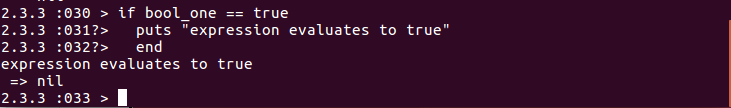We've passed the boolean expression "bool_one == true" as an evaluator of the if statement. But we can also do it like this.

```2.6.8 :007 > if 5 !=7
2.6.8 :008?>   puts "expression evaluates to true"
2.6.8 :009?>   end```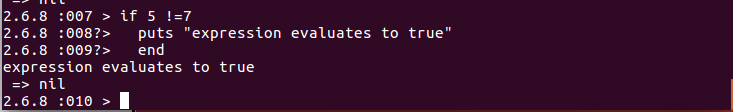This time we're passing a boolean expression directly in the if statement evaluator. Note that we didn't need the final evaluation of "== true" because the current expression evaluates that for us. As we can see, there are slight differences when we pass the boolean expression inside a boolean variable and when we pass the boolean expression directly. The usage of one or the other will depend on the use case. This last case is more straightforward than the last one.

But the significant advantage of the boolean variables (and variables in general) is that we have just one place (in theory) where we can change values without affecting the logic developed inside the program. In addition, a variable means that the value inside will change at any moment, so we can have dynamic code with it. In contrast, if we pass values directly, these values won't change dynamically shortly.

So, we have to make the change manually, which is not a good practice.

# Other Comparison Operators

So far we’ve seeing just two comparison operators

• * Equal: ==
• * Not equal: !=

Now let's see others, like
• * Greater than: >
• * Less than: <
• * Greater than or equal to >=
• * Less than or equal to <=

Let's do some examples.

```2.6.8 :010 > 5 > 2
=> true
2.6.8 :011 > 5 < 2
=> false
2.6.8 :012 > 10 >= 10
=> true
2.6.8 :013 > 10 <= 10
=> true
2.6.8 :014 > 20 >= 10
=> true
2.6.8 :015 > 20 <= 10
=> false ```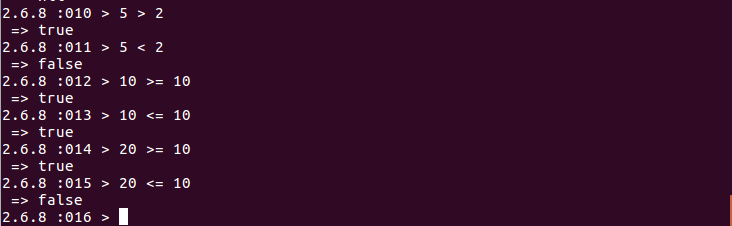I guess that these operations are precise at this point of the post. We're executing boolean expressions directly in our IRB console. With the power of Ruby, we can compare Integer values with these comparison operators.

# Logical operators

Often the conditionals you will evaluate will require more than two Boolean expressions. You can do this with Boolean operators (also called logical operators). Comparison operators allow us to assert the equality of a statement with Ruby. For example, we can argue whether two values or expressions are equal with ==, or whether one value is more significant than another with >.

However, there are scenarios in which we must assert whether multiple values or expressions are valid. In Ruby, we can use logical operators to make these assertions.

The Boolean operators are:

• * And: in Ruby, the syntax is "&&"
• * Or: in Ruby, the syntax is "||"

I know it is a bit weird, but let's explain this more in detail because it is critical. Let's suppose that you want to give access to a user on your webpage, and it depends on the country and the age. Accordion to this use case, we have to evaluate the next conditionals

1. 1. If a user belongs to an authorized country
2. 2. And
3. 3. If the user has more than a given age.

1. We can see this expression as quickly as the text we expressed above. The first line needs to be evaluated to be accurate, AND the second line also needs to consider true. If both are true, then the user can access the webpage. Let's extrapolate this to a Ruby code.

```2.6.8 :001 > country = "Portugal"
=> "Portugal"
2.6.8 :002 > age = 25
=> 25
2.6.8 :003 > if country == "Portugal" && age >= 18
2.6.8 :004?>   puts "user can enter the webpage"
2.6.8 :005?>   end
user can enter the webpage```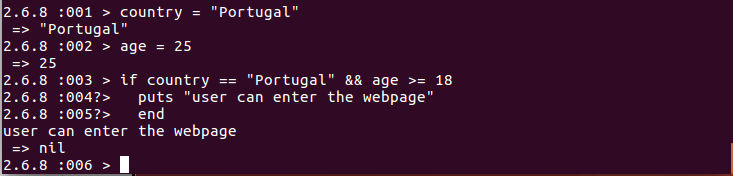Easy peasy!

First, we've assigned two variables: "country" and "age," and give them an initial value to each one of them. Then we call the if statement evaluating first the country and then evaluating the age. Both boolean expressions need to be true to execute the code, which is the case. Now let's give access to people from Portugal OR Spain, who have to be older than 18 years. How can we do this?

We've just to change the if statement like so

```2.6.8 :006 > if (country == "Portugal" || country == "Spain") && age >= 18
2.6.8 :007?>   puts "user belongs to an authorized country and is older than 18"
2.6.8 :008?>   end```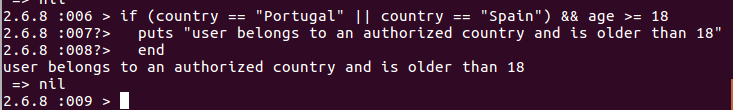Now we’re evaluating 3 boolean expressions

• * country == "Portugal"
• * country == "Spain"
• * age >= 18

The difference here is that the && and || evaluate them accordingly and in order (given the parenthesis). The OR operator is also easy to understand; the whole expression will be true if any of them is true. Let's do an example, where are you from? From Portugal or from Spain? If you are from another country, the result is false. But, on the other hand, the output will be true if you're from these two countries.

# Truth table

Time to see the following concept: truth table. A truth table is a mathematical table used in logic, specifically with Boolean algebra, boolean functions, and propositional calculus.

Which sets out the functional values of logical expressions on each of their applicable arguments, that is, for each combination of values taken by their logical variables. In particular, truth tables can be used to show whether a propositional expression is true for all legitimate input values, that is, logically valid.

As always, theory can be more complicated than reality is. Nevertheless, the keys take aways are:

• * If you're evaluating expressions with && (and), all expressions need to be true to get an output equal to true. Otherwise, the result will be false
• * If you're evaluating expressions with || (or) if just one expression is true the output will be equal to true. Otherwise, the result will be false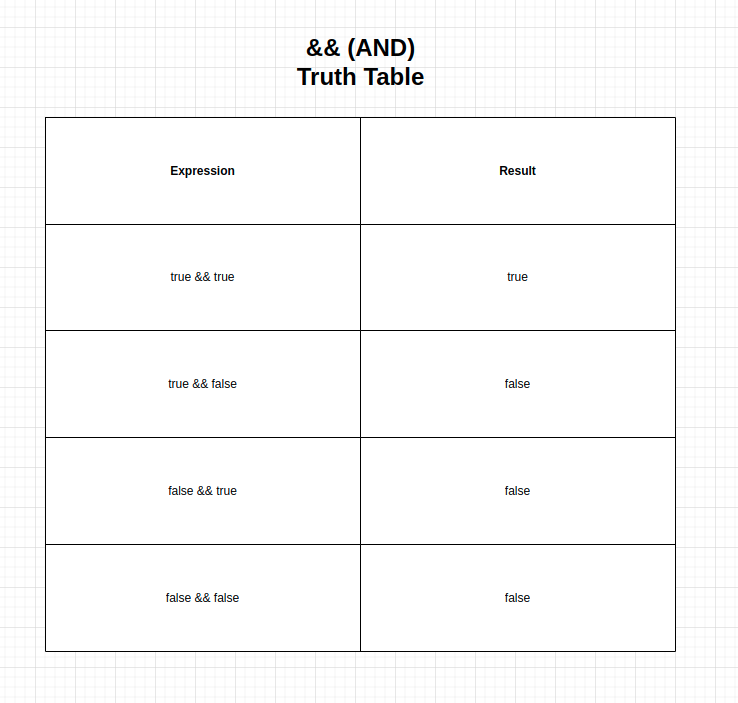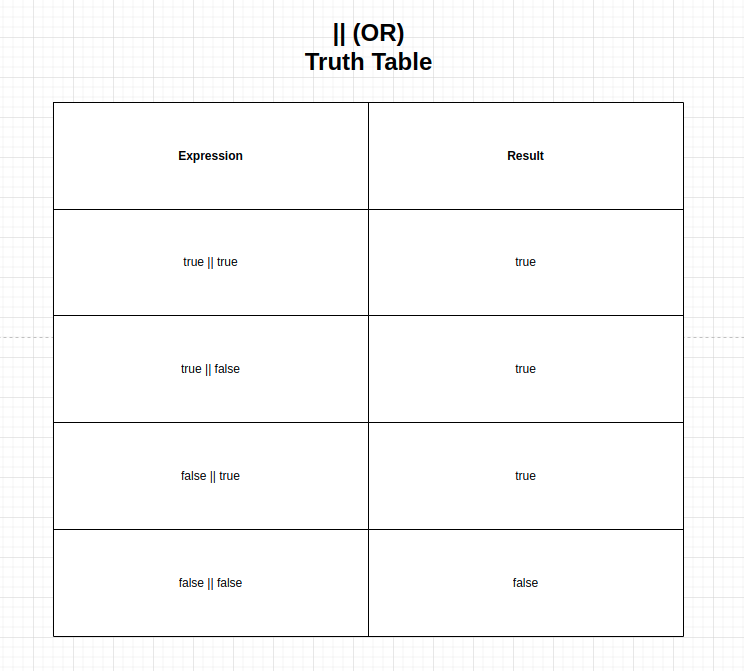Let's do some coding with this new knowledge.

```2.6.8 :009 > (1 + 1 == 2) && (2 + 2 == 4)
=> true
2.6.8 :010 > (1 + 1 == 2) && (2 < 1)
=> false
2.6.8 :011 > (1 > 9) && (5 != 6)
=> false
2.6.8 :012 > (0 == 10) && (1 + 1 == 1)
=> false ```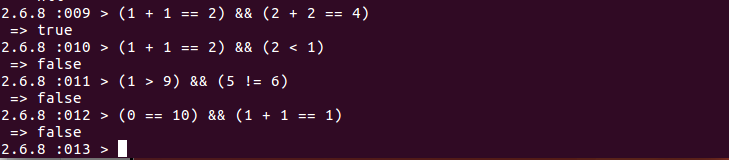It's a good exercise if you try to figure out why we are getting these results. But, again, remember to consider the parenthesis and do the analysis after and before the Logical Operator.

Let's see more examples.

```2.6.8 :018 > (3 == 8) and 5 == 5
=> false
2.6.8 :019 > true || (3 + 4 == 7)
=> true
2.6.8 :020 > (1 - 1 == 0) || false
=> true
2.6.8 :021 > (2 < 0) || true
=> true
2.6.8 :022 > (3 == 8) || (3 > 4)
=> false ```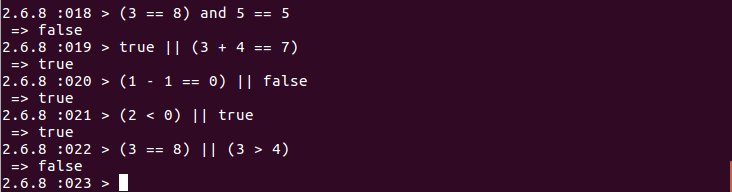Can you figure out why these results? Remember to do this by yourself. The key to understanding how this works is like this:

• * Start doing your mental operations from left to right
• * Remember the rules of && and || that basically says. If && has a false, automatically will be evaluated to false. If || has true, it automatically will be considered true
• * Group by each parenthesis
• * Finally execute the && or the ||

All right, we're almost done before with control flow and conditionals. So, let's see the following concept "elsif statement."

# Elsif statement

Elsif stands for "Else if." The Else If statement checks another condition after the previous conditionals if they are not met.

Example

```if <condition_1>
# execute this code if <condition_1> is met
elsif <condition_2>
# execute this code if <condition_1> is NOT met, but condition <condition_2> is met
elsif <condition_3>
# execute this code if <condition_1> and <condition_2> is NOT met, but condition <condition_3> is met
end```

Let's do the code inside our IRB.

```2.6.8 :040 > country = "Portugal"
=> "Portugal"
2.6.8 :041 > if country == "Portugal"
2.6.8 :042?>   puts "You live in Portugal. That's nice!"
2.6.8 :043?>   elsif country == "Austria"
2.6.8 :044?>   puts "You live in Austria. That's awesome!"
2.6.8 :045?>   end
You live in Portugal. That's nice```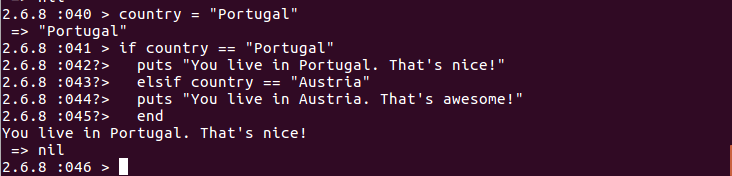# Else statement

Once you start including a lot of IF clauses, the code starts to become unreadable and unintuitive. For this, there are other tools we can use to control the flow. The else clauses allow us to describe what we want to do if the conditions of the previous clause are not met. The else clauses always appear in conjunction with the if-clauses.

```if <condition_1>
# execute this code if <condition_1> is met
elsif <condition_2>
# execute this code if <condition_1> is NOT met, but condition <condition_2> is met
elsif <condition_3>
# execute this code if <condition_1> and <condition_2> is NOT met, but condition <condition_3> is met
else
# execute this code if none of the above are met
end```

Let's do the code inside our IRB.

```2.6.8 :046 > country = "Portugal"
=> "Portugal"
2.6.8 :047 > if country == "Portugal"
2.6.8 :048?>   puts "You live in Portugal. That's nice!"
2.6.8 :049?>   elsif country == "Austria"
2.6.8 :050?>   puts "You live in Austria. That's awesome!"
2.6.8 :051?>   else
2.6.8 :052?>   puts "You live in another country, but we don't know which one :("
2.6.8 :053?>   end
You live in Portugal. That's nice!```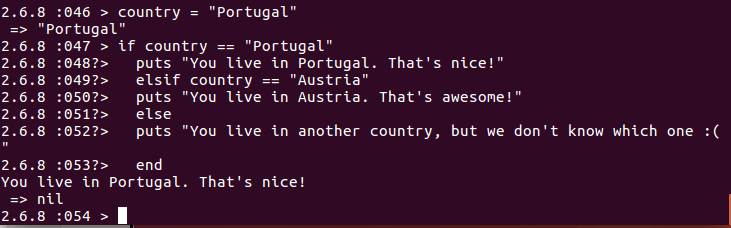# Executing code outside IRB

Seems like our code inside our IRB console is starting to grow, and we cannot differentiate the sentences very well. So, following this blog post about Text Editors, let's do all of this again from Visual Studio Code and execute it with the terminal or console.

First, we create a new file called index.rb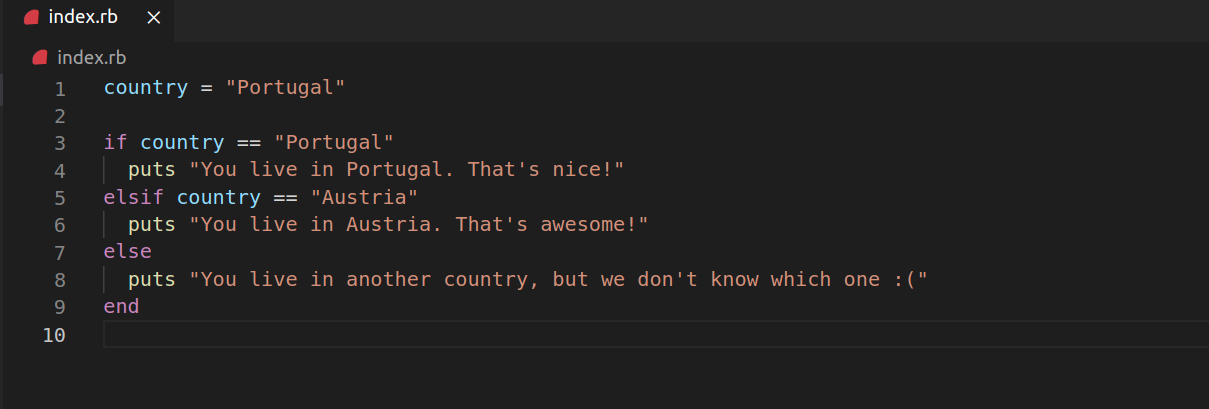Then we access it from the terminal, and then we execute the command ruby <filename>.rb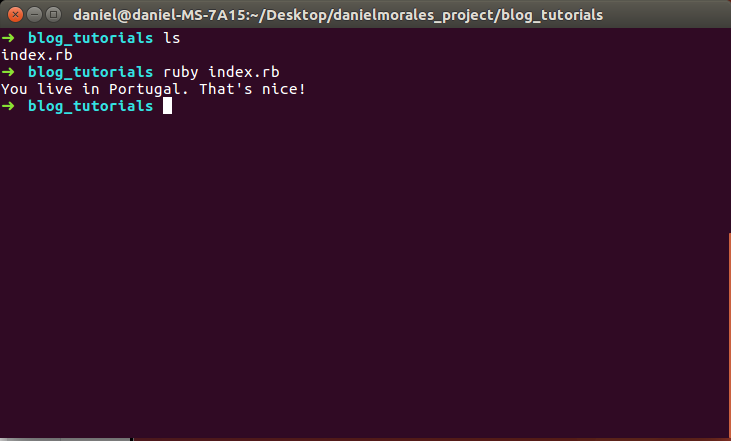Now let's make it more dynamic. But, first, let's take the user input, as we've learned in the previous post.

```puts "Please enter the country where you currently live: "
country = gets.chomp

if country == "Portugal"
puts "You live in Portugal. That's nice!"
elsif country == "Austria"
puts "You live in Austria. That's awesome!"
else
puts "You live in another country, but we don't know which one :("
end```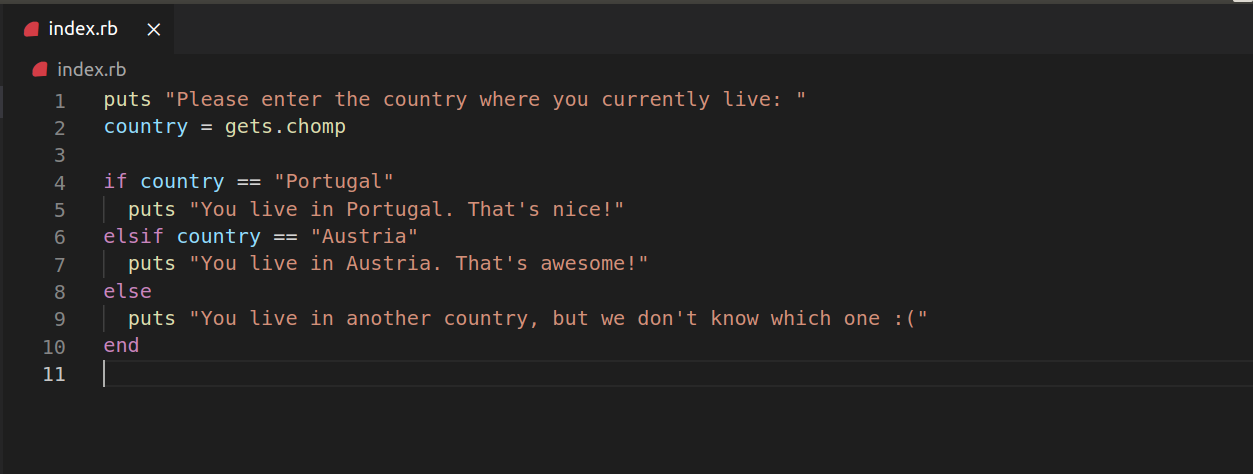Now let's execute it from the console.Can you figure out the changes we made? We've now a more dynamic code, requesting user inputs. Our program is going to the control flow, asking questions with the boolean expressions and then printing the result according to that evaluation. Pretty nice, no?

I hope you've learned a lot about conditionals. These are the main blocks of any programming language. Later will be doing more exercises to practice this newly acquired skill.